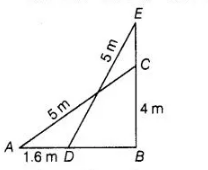# A 5m long ladder is placed leaning towards

Question:

A 5m long ladder is placed leaning towards a vertical wall such that it reaches the wall at a point 4 m high. If the foot of the ladder is moved 1.6 m

towards the wall, then find the distance by which the top of the ladder would slide upwards on the wall.

Solution:

Let AC be the ladder of length 5 m and BC = 4 m be the height of the wall, which ladder is placed. If the foot of the ladder is moved 1.6 m towards

the wall i.e, AD = 1.6 m, then the ladder is slide upward i.e. ,CE = x m.

In right angled ΔABC,

$A C^{2}=A B^{2}+B C^{2}$ [by Pythagoras theorem]

$\Rightarrow$ $(5)^{2}=(A B)^{2}+(4)^{2}$

$\Rightarrow$ $A B^{2}=25-16=9 \Rightarrow A B=3 m$

$\therefore$ $D B=A B-A D=3-1.6=1.4 \mathrm{~m}$In right angled $\triangle E B D$,  $E D^{2}=E B^{2}+B D^{2}$  [by Pythagoras theorem]

$\Rightarrow \quad(5)^{2}=(E B)^{2}+(1.4)^{2}$ $[\because B D=1.4 \mathrm{~m}]$

$\Rightarrow$ $25=(E B)^{2}+1.96$

$\Rightarrow$ $(E B)^{2}=25-1.96=23.04$

$\Rightarrow$ $E B=\sqrt{23.04}=4.8$

Now, $E C=E B-B C=4.8-4=0.8$

Hence, the top of the ladder would slide upwards on the wall at distance 0.8 m.